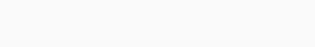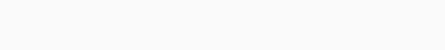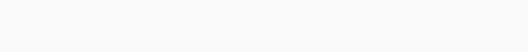# Solved – Interpretation of model comparison with random slopes using lmer in R

I have a large data set with repeated measurements of same blood value (co) (2 to 7 measurements per patient). Each measurement is coupled with time which is the time interval between surgical operation and blood level measurement.

My aim is to show that this blood value correlates positively with time.

Blood level measurements are highly skewed to right and hence I am using a log-transformation and linear mixed effect regression model (lmer in lme4 package).

At first I assumed random intercepts among patients. I constructed a null model and model with time as independent.

``fit0<-(lmer(lgco~(1|id),data=mebhr)) fit1<-(lmer(lgco~time+(1|id),data=mebhr)) anova(fit0,fit1) refitting model(s) with ML (instead of REML) Data: mebhr Models: fit0: lgco ~ (1 | id) fit1: lgco ~ time + (1 | id)      Df    AIC    BIC  logLik deviance  Chisq Chi Df Pr(>Chisq)     fit0  3 200.44 213.16 -97.219   194.44                              fit1  4 189.62 206.59 -90.811   181.62 12.815      1  0.0003438 *** --- Signif. codes:  0 ‘***’ 0.001 ‘**’ 0.01 ‘*’ 0.05 ‘.’ 0.1 ‘ ’ 1 ``

Ok, so I have an empty model and model with independent variable.Adding covariate time in my model improves it significantly and also the graphical explanation is clear.

Fixed slopes, however, are not reasonable in my data, so I should use random slopes.

``fit0<-(lmer(lgco~(time|id),data=mebhr)) fit1<-(lmer(lgco~time+(time|id),data=mebhr)) anova(fit0,fit1) refitting model(s) with ML (instead of REML) Data: mebhr Models: fit0: lgco ~ (time| id) fit1: lgco ~ time + (time | id)      Df    AIC    BIC  logLik deviance  Chisq Chi Df Pr(>Chisq)    fit0  5 190.15 211.36 -90.076   180.15                             fit1  6 182.06 207.51 -85.029   170.06 10.094      1   0.001487 ** --- Signif. codes:  0 ‘***’ 0.001 ‘**’ 0.01 ‘*’ 0.05 ‘.’ 0.1 ‘ ’ 1 ``

At this point I dont understand my model equations. Graphical outputs for fit0 and fit1 are as follows:For the fit1 the model equation is:Why the lines in fit0 have non-zero slopes? What are they and what is the equation in that case? Also I dont understand how should I clarify the change in model fit? In the case of only random intercepts I can state that "adding fixed factor beta1 to model improves it significantly". What would be the equal statement in the case of random slopes?

Contents

1. The lines at `fit0` have non-zero slopes because you allow for random slopes in your model specification (`(time|id)`).

2. When you exclude `time` you essentially remove the term \$beta_1\$ from your model. In general, as with intercepts, it makes sense to include a model-wise slope. When you remove the fixed-effect slope (here `time`), you make the assumption that there is no `time` effect to your response variable (`lgco`) that is common to all subjects (i.e., \$beta_1 = 0\$). You are almost destined to allocate any common variance from fixed effects to the random effects in that way (and subsequently increase their significance as well as alter their values).

3. I believe you do not have to say anything. You can safely conclude by simply hinting that any common effects should be captured by that term. Your expert knowledge dictates that something should be in the model and you therefore correct for it. Most of all, your model reflects your modelling assumption and not what your \$p\$-values, \$F\$-test, etc., tell you about your model. 🙂

4. Some further thoughts:

I. You might want to consider to have uncorrelated random intercepts and slopes too (e.g., `lmer(... + (1|id) + (0+time|id)`). This is a more restrictive assumption but it would allow you to interpret your resulting random effects coefficients independently.

II. Bootstrap your model (e.g., `bootMer`), or at least use an educated AIC (e.g., see here and here for details) for model selection too.

Rate this post